# How to make formulas in Excel

The tutorial explains how to write formulas in Excel, beginning with very simple ones. You will learn how to create a formula in Excel using constants, cell references and defined names. Also, you will see how to make formulas using the function wizard or enter a function directly in a cell.

In the previous article we've started to explore a fascinating word of Microsoft Excel formulas. Why fascinating? Because Excel provides formulas for almost anything. So, whatever problem or a challenge you are facing, chances are that it can be solved by using a formula. You just need to know how to make a proper one :) And it is exactly what we are going to discuss in this tutorial.

For starters, any Excel formula begins with the equal sign (=). So, whatever formula you are going to write, begin by typing = either in the destination cell or in the Excel formula bar. And now, let's have a closer look at how you can make different formulas in Excel.

## How to make a simple Excel formula by using constants and operators

In Microsoft Excel formulas, constants are numbers, dates or text values that you enter directly in a formula. To create a simple Excel formula using constants, just do the following:

• Select a cell where you want to output the result.
• Type the equal symbol (=), and then type the equation you want to calculate.
• Press the Enter key to complete your formula. Done!

Here is an example of a simple subtraction formula in Excel: `=100-50`

## How to write formulas in Excel using cell references

Instead of entering values directly in your Excel formula, you can refer to the cells, containing those values.

For example, if you want to subtract a value in cell B2 from the value in cell A2, you write the following subtraction formula: `=A2-B2`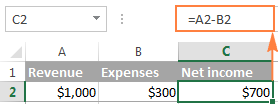When making such a formula, you can type the cell references directly in the formula, or click the cell and Excel will insert a corresponding cell reference in your formula. To add range reference, select the range of cells in the sheet.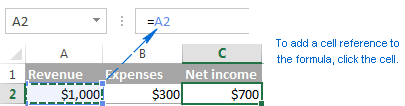Note. By default, Excel adds relative cell references. To switch to another reference type, press the F4 key.

A big advantage of using cell references in Excel formulas is that whenever you change a value in the referred cell, the formula recalculates automatically without you having to manually update all the calculations and formulas on your spreadsheet.

## How to create an Excel formula by using defined names

To take a step further, you can create a name for a certain cell or a range of cells, and then refer to that cell(s) in your Excel formulas by simply typing the name.

The fastest way to create a name in Excel, is to select a cell(s) and type the name directly in the Name Box. For example, this is how you create a name for cell A2: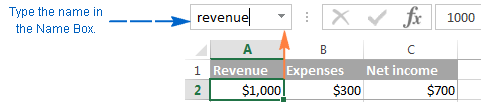A professional-like way to define a name is via the Formulas tab > Defined names group or Ctrl+F3 shortcut. For the details steps, please see creating a defined name in Excel.

In this example, I've created the following 2 names:

• revenue for cell A2
• expenses for cell B2

And now, to calculate the net income, you can type the following formula in any cell on any sheet within the workbook in which those names were created: `=revenue-expenses`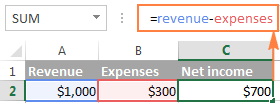In the same manner, you can use names instead of cell or range references in arguments of Excel functions.

For example, if you create the name 2015_sales for cells A2:A100, you can find a total of those cells by using the following SUM formula: `=SUM(2015_sales)`

Of course, you can get the same result by supplying the range to the SUM function: `=SUM(A2:A100)`

However, defined names make Excel formulas more understandable. Also, they can significantly expedite creating formulas in Excel especially when you are using the same range of cells in multiple formulas. Instead of navigating between different spreadsheets to find and select the range, you just type its name directly in the formula.

## How to make Excel formulas by using functions

Excel functions are nothing else than predefined formulas that perform the required calculations behind the scene.

Each formula begins with an equal sign (=), followed by the function name and the function arguments entered within the parentheses. Each function has specific arguments and syntax (particular order of arguments).

For more information, please see a list of the most popular Excel functions with formula examples and screenshots.

In your Excel spreadsheets, you can create a function-based formula in 2 ways:

### Create a formula in Excel by using the Function Wizard

If you do not feel very comfortable with Excel spreadsheet formulas yet, the Insert Function wizard will give you a helpful hand.

#### 1. Run the function wizard.

To run the wizard, click the Insert Function button on the Formulas tab > Function Library group, or pick a function from one of the categories: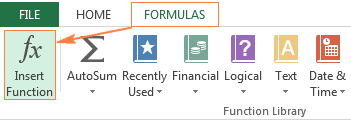Alternatively, you can click the Insert Function button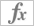to the left of the formula bar.

Or, type the equal sign (=) in a cell and pick a function from the drop-down menu to the left of the formula bar. By default, the drop-down menu displays 10 most recently used functions, to get to the full list, click More Functions...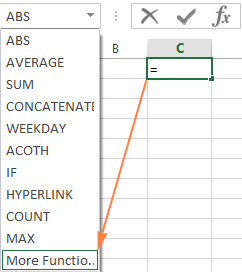#### 2. Find the function you want to use.

When the Insert Function wizard appears, you do the following:

• If you know the function name, type it in the Search for a function field and click Go.
• If you are not sure exactly what function you need to use, type a very brief description of the task you want to solve in the Search for a function field, and click Go. For example, you can type something like this: "sum cells", or "count empty cells".
• If you know what category the function belongs to, click the small black arrow next to Select a category and choose one of the 13 categories listed there. The functions belonging to the selected category will appear in the Select a function

You can read a short description of the selected function right under the Select a function box. If you need further details regarding that function, click the Help on this function link near the bottom of the dialog box.

Once you've found the function you wish to use, select it and click OK.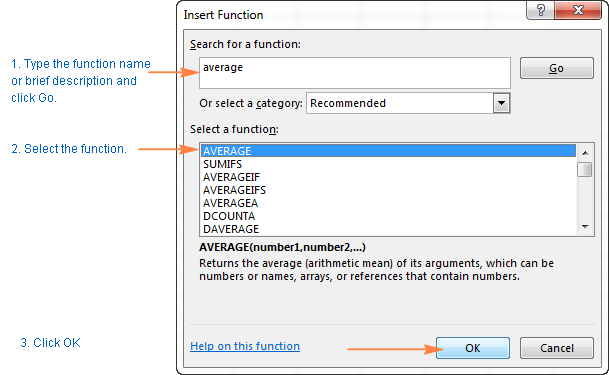#### 3. Specify the function arguments.

In the second step of the Excel function wizard, you are to specify the function's arguments. Good news is that no knowledge of the function's syntax is required. You just enter the cell or range references in the arguments' boxes and the wizard will take care of the rest.

To enter an argument, you can either type a cell reference or range directly into the box. Alternatively, click the range selection icon next to the argument (or simply put the cursor into the argument's box), and then select a cell or a range of cells in the worksheet using the mouse. While doing this, the function wizard will shrink to a narrow range selection window. When you release the mouse button, the dialog box will be restored to its full size.

A short explanation for the currently selected argument is displayed right under the function's description. For more details, click the Help on this function link near the bottom.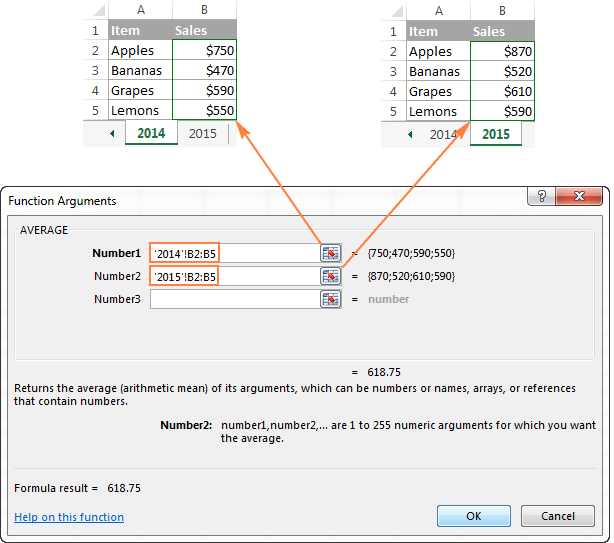Excel functions allow you to perform calculations with cell residing on the same worksheet, different sheets and even different workbooks. In this example, we are calculating the average of sales for 2014 and 2015 years located in two different spreadsheets, which in why the range references in the above screenshot include the sheet names. Find more about how to reference another sheet or workbook in Excel.

As soon as you've specified an argument, the value or array of values in the selected cell(s) will be displayed right to the argument's box.

#### 4. Complete the formula.

When you have specified all the arguments, click the OK button (or just press the Enter key), and the completed formula is entered into the cell.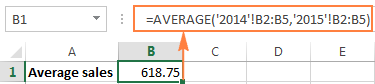### Write a formula directly in a cell or formula bar

As you've just seen, creating a formula in Excel by using the function wizard is easy, thought it's quite a long multi-step process. When you have some experience with Excel formulas, you might like a faster way - typing a function directly into a cell or formula bar.

As usual, you start by typing the equal sign (=) followed by the function name. As you do this, Excel will perform some kind of incremental search and display a list of functions that match the part of the function's name you've already typed: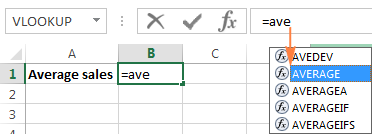So, you can either finish typing the function name on your own or select from the displayed list. Either way, as soon as you type an opening parenthesis, Excel will show the function screen tip highlighting the argument you need to enter next. You can type the argument in the formula manually, or click a cell (select a range) in the sheet and have a corresponding cell or range reference added to the argument.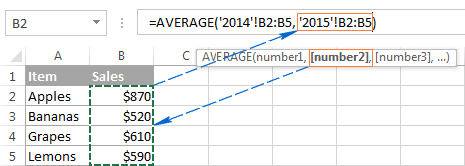After you've input the last argument, type the closing parenthesis and hit Enter to complete the formula.

Tip. If you are not quite familiar with the function's syntax, click the function name and the Excel Help topic will pop-up right away.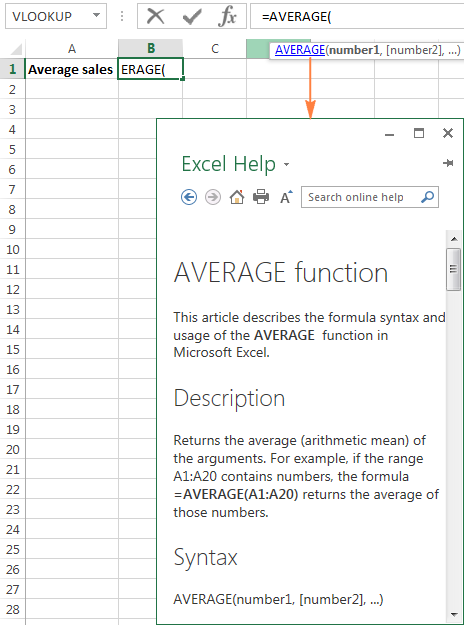This is how you create formulas in Excel. Nothing difficult at all, is it? In the next few articles, we will continue our journey in the intriguing realm of Microsoft Excel formulas, but those are going to be short tips to make your work with Excel formulas more efficient and productive. Please stay tuned!

## You may also be interested in

### 80 comments to "How to make formulas in Excel"

1.Hemanth says:

Hi, i have a question. The above post says about the existing formulas in Excel.
In Excel 2021, there is custom formula option using java script and saving the file.
Is there any way to insert custom formula in MS Excel 2016.
For example, I require numbers to be presented in Text as well

Cell A1: 2000
Cell A2: Two Thousand

There is option for the same in 2021 version of MS Excel "=Spellnumber" but, how to do the same in 2016 version, without VBA and macro enabled workbook, to be specific

2.Ayuba Sunday says:

Hi kindly help me I intent calculating an undisclosed turnover in other to arrive at the right tax by using a formula from the excel

3.Tarun says:

45/80+34/40+23/80 which formula in used in excel
Average in same and percentage

4.churchill says:

I need a formula,

Eg

Customer name Achieve QTY Basic value
A 5 150
B 5 150
A 5 150
C 15 450
B 5 150
A 5 150

this is the details here i need to take customer name "A" first "10" basic value.
Result
A 5+5 150+150

A 10 300
like this
any one can help me.

•Alexander Trifuntov (Ablebits Team) says:

Hello!
Sorry, it's not quite clear what you are trying to achieve. Customer A is recorded 3 times, but the result is counted in two. How many values do you need to calculate?

•Hemanth says:

Hello, if you haven't yet got answer for your question... Use a =SUMIF formula. It gives you options for the above.

5.Pallab Datta says:

An amount of 1000 need to be increased by 500 every 3 yrs. from 01.04.2021 till 31.12.2026. Kindly frame a formula

6.tanu says:

wthat is the formula of 120-68.55% in excel or k9-19%

7.RISHAMA says:

HI! GOOD DAY
I NEED A TO CALCULATE POSITION IN EXCEL. THANK YOU.

8.Weam says:

Hello, i am working on an excel sheet i need help
I want to know who are taking medicine in a village
I fill a table column by the name of the villagers and another by medicine and the 3rd is insurence
The medicine and indusrence column only include yes or no
How could i make on another page only the name of the villager display automatically if he takes medicine and doesn't have insurence?

9.zubair says:

column-A , column-B
1
2
3
4
5
6
7
8
9
10
11
12
13
14
15........
and serial go more then 4000,
so i want a print out on paper size A4, like this:-
column-A , column-B , column-C, column-D
1 , 11 , 21 , 31
2 , 12 , 22 , 32
3 , 13 , 23 , 33
4 , 14 , 24 , 34
5 , 15 , 25 , 35
6 , 16 , 26 , 36
7 , 17 , 27 , 37
8 , 18 , 28 , 38
9 , 19 , 29 , 39
10 , 20 , 30 , 40 and so on

10.SANJAY says:

(2+2)/2*2 =?

•Svetlana Cheusheva says:

4 :)

11.hussain says:

Is it possible to use a formula to make a statement using a spreadsheet including many data.
I just want extract some data from sheet

12.JOAO ROBERTO PIRES DE CAMPOS NETO says:

Hi.
How can I insert what is written in a cell inside a formula of other cell?
For instance:
Cell A1:
Cell B1
an in another cel is writen AAPL

13.HIMANSHU says:

i need a formula

if "A2" value >100 then (*"C2") other then (*"A2*C2/100")

•Mary Trifuntova (Ablebits Team) says:

Hello Himanshu,

=IF(A2>100,B2*C2,B2*A2*C2/100)

14.Anthony says:

How can I create a formula that take a value entered and add the value from another cell and display it in the active cell?

15.Janet says:

I need a formula where a word equals a percentage. Yes in Cell A2 = 25%. Yes in Cell B2 =10% and so on

16.Nagar Mal says:

there is date of birth in a cell in figures how can we change it in words in another cell. Pl. let me know the formula.

17.Kyla says:

Hi, I am creating a spreadsheet mostly using the edate function to calculate how far in the future an activity should occur. Initially there is no date but when I drag down the formula that will give me a date, it fills in a date that ends in 1900. I don't want any date to show until the initial date is supplied. How do I write the fromula to include the edate function and be blank. This is the formula for the date to calculate[@[1st Quarter Student Eval Date]] (edate) and this is the formula for a cell to remain blank: =IF(OR(ISBLANK(D5)), "", D5+S5)

18.Barton Lewis says:

Hello, I have a spreadsheet with height and width values of artworks. I want to sell those at different print sizes, so I would like to be able to enter a formula that calculates the width and height of each at 75% and 50%. Is this possible? Thank you.

19.NARESH BHOI says:

Dear Sir ,

F@30% =B

20.mike says:

Hi
Imagine I have two firms. the market size is 1000 and one firm has a price of \$5 and one of \$7. How do we split market share? Next one firm spends \$30 on advertaing with a price of 45 and the other spends \$10 (price \$7). How do we combine the two variable price and advertising spend. and what about more variables. Is there a simple equation?

21.Yasir says:

I want to make a shop drawings log , need to calculate total number of drawings, Approval & comments by alphabetic words (exp. A for approval C for to be revised.)

can some one help me please

22.Rinki says:

How can I add this no in excel
110abc + 12

23.Umair says:

Dear
i am paying bounce on producton to my employees working in my factory.and i want a formula for this plz help me if production is 400,000 then bounce is 100 or if production is 425,000 then bounce is 150 or as below
Production Bounce
400,000 100
425,000 150
450,000 200
475,000 250
500,000 400

24.Alex says:

Hi,

* I had been looking for a new formula which"ll feed me with my kinda stuff.
After several searches and experiments, i didn't stopped at nothing.
I'm still in a thirst for it.

* Its hard to explain, but lets see if i'm a good teacher

* Consider a single cell containing a paragraph and there are "n" number of cells and what i need is to extract numbers or alpha's or both of a specific term which is repeated in each cell.

* For Example, Consider 2 cells of paragraph containing Phone numbers
In Cell 1 - ABCDEFGHIJKLMNOPQRSTUVWXYZ Ph no. 123456789 asdfasdfasdf
In Cell 2 - ABCDEFGHIJKLMNOPQRSTUVWXYZ Ph no. 987654321 asdfasdfasdf
and the formula i need is will be like ...

=SEARCH("Ph no. ") and the main thing i'm looking to add with this is... i need all the numerics or alpha's set (till the position in which it is NOT seperated by a space or comma) to be derived to the cell i want

* And now consider Cell 3 to be a place where i am entering the formula i need from Cell 1 and same with Cell 4 to be a place where i am entering the formula i need from Cell 2

* With refence to above example Cell 3's visible data what i need is should be
"Ph no. 123456789"
* With refence to above example Cell 4's visible data what i need is should be
"Ph no. 987654321"

* Irrespective of the position where the " Ph. no " in a cell is placed

* I know you might think im crazy LoL but it'd really be helpful to me if u help me in this..

25.Madilin says:

Hi I need help
I would like to split 1.5x 2.5 which is in the same column.
I want data to be in different column or seperated to be
1.5
2.5
How can I do that

•Mary Trifuntova (Ablebits Team) says:

Thank you for contacting us.

If we understand your task correctly, please have a look at our Split Cells add-in. It can help you separate your text into multiple columns or rows at a glance. The add-in is included in our Text Toolkit for Excel that contains 8 useful add-ins to manage text data in Excel (remove extra spaces, substrings and non-printable characters, change case, split cells, etc.).

You can install a fully functional 7-day trial version of Text Toolkit and see if it works for you. Here is the direct download link.

Don’t hesitate to contact us again if we can help in any way.

26.Carley Onofreo says:

I am trying to find a formula to figure out 6% sales tax.
Sales ÷tax = expenses

Thank you

•Doug says:

Carley:
I think what you want is the formula:

27.Mushtak Ahmed says:

I am trying to write the following formula in excel

x=100[((4a+b+c+d)e)-6]/254 where a, b, c, d, e have numerical values in various cells.

Can some help !

Many thanks

•Doug says:

Mushtak:
I think this will provide the result you're after:
=100*(((4*(A1)+B1+C1+D1)*E1)-6)/254
Note the A1, B1, C1, D1 and E1 are cell addresses that you can modify.
Note the asterisk Excel uses to signify multiply.
Note there are no square brackets.

28.virendra says:

if i want that no.enter in the cell should be divided by 1000 and round upto one decimal place. like 6145 should be converted into 6.1
what will be the formula .

•Doug says:

Virendra:
The simplest way to get what you want is to apply some arithmetic and then formatting a cell as a number to display one digit. If you want to do this "automatically" you'll need to build some VBA code and that's beyond the scope of this blog. I'll show you the easy method.
Let's say your number is in cell A2. Maybe it is 6145.
First, in cell B2 enter =A2/1000.
Next you'll format the cell B2 as a number with one decimal place.
To format the cell, right click on B2, choose Format Cells, then choose Number, then select 1 decimal place. Click OK.
The number displayed is "6.1".
Some arithmetic, some formatting and you're home.

29.virendra says:

if i want that no.enter in the cell should be divided by 1000 and round upto one decimal place. like 6145 should be converted into 6.1
what will be the formula .

30.Tina says:

Hi, Can anyone tell me how to program this: I want h31 to be multiplied by 3.5% that i have in G32 and want the total of h31 g32 to total in h32.

31.John T says:

In developing a formula to solve a mortgage question with time/term being the unknown, how do I use PMT & 'What If' to create it??? Or is there a better way??? ie:
PV: \$100,000
I: 5.0%
Pmyt: \$536.83
FV: \$00
Solve for Unknown 'N': ???

Thank you for your insight & assistance, jt

32.Abdul Rasheed says:

hellow i need some help!

1) In regards with the formulas of Excel can i create my own formulas and How?

2) I need to Insert Particular Page No of a Cell Located in Different Sheets
for which i am unable to find the formulas in excel
can i get some help

will be Thankful for that

33.Andrew says:

Hi I’m trying to find a formula or combined for predicting a future date from a set date depending on a cell value in another work sheet.
Example:
01/01/2018 = future date?
Based on a score of 1 with an argument of next date is 20 days when a score is between 1-5.
6-10 =15 days

34.Amit K says:

Hello,
I have a calculation, which needs to be integrated in sheet. I am unable to figure out the formula for it -
EG:
Freight (10 X 8)
(+) 200
Result of above * 16%
(+) 50
Result of above * 18%

35.McQueen says:

Hellow
Write an Ms Excel Formula for each of the following measures:
a)the statistical range of all values in cells K2,L3,M4 and the cell range AZ4:BB6
b)the interest given the principle in A2,the annual interest rate in A3 and the number of years in A4,where interest is compounded every two months.
Your kind response in help will be much appreciated.

36.MEHRDAD says:

Hi
How can i write equation in excel?

37.Mary says:

I need to have a date to mail out notices 90 days after a date in another column depending on if another column says needs testing.

Is there a formula to do this, or do I just do this myself?

38.Andrew says:

hi, i have an issue writing a formula and am not sure if it could be done, but i would love to here your input.

i have a spreadsheet made up for my work team and need a formula to turn particular arguments into a number.

i have 5 guys on a team.
when they come in for a shift i use a date picker to select the day which includes an abbreviation of the day i.e (wed)

i also have to take into account if its a night shift or day shift so i fill the cell colour red for night and blue for day.

i also need to add to these arguments a figure regarding to the day of the week (wed) and the colour of the cell (day / night shift).

unless there is a date picker around i could modify so it would ask what shift was done on the day/date selected.

so for example. if i selected a cell the right click and choose a date from the date picker, the formula would then calculate a figure answer with the day of week and shift. shifts have different values.

thanks your help will be greatly appreciated.

regards Andrew.

39.Vijay Pawar says:

Hi,
I'm looking for a formula that I have seen on google (mostly from your blog) but now I forgot and I searched lot on google but I didn't find what I'm looking for.
In my formula I want to change/increase only row number/reference by '1' not column number/reference.
***Not cell value
like this
A1
A2
A3
A4

40.Morris Edwards says:

Thanks for sharing such type of informative post with us. Keep it up.

41.Richard Amoh says:

please help me to find formulas that will help me to manage my whole sales and retail agro.chemical shop.

42.Richard Amoh says:

please I need a formula that will help me to manage my whole sale and retail shop . in fact I'm wondering which formula to use.

43.Ved Prakash Sahu says:

44.Ved Prakash Sahu says:

I want a unique formula to create different formulas
Example

SUM .......
Average
Count

I want a unique formula at the place of dots for all three calculations(after dragging in all three cells).
it means "reference of the formula not value or range"
Is it possible?

45.lakshmi says:

good useful

46.aqsa says:

Hi..
I have a task to use concatenate function with if function to solve simple arithmetic questions and I have to give ans with the step just like '5*6=30' how can I so this

47.Kochurani says:

I am having trouble writing a formula and have become extremely frustrated. I know .17 is 17% but is written as 1.17 in the excel formula. Is this correct? Should I be using .17 (which makes my mark-up off by a lot)or 1.17? If I am to use 1.17 why? I do not understand. PLEASE HELP ME!!

Formula

=(gross pay + bonus)* 1.17)+ stipend + cost of insurance

Trying to get the total w/ a 17% burden

48.Baiju says:

Hi Svetlana,

Need a formula based on below criteria:
I have two cells, A and B,
if the difference btw A & B is less than 6 or equal to 6, "No Change"
if greater than 6 and less than 9, "Slight Change"
if greater than 10 "Significant Change"

Also the formula should override the difference with positive numbers, even the B is larger than A, vice-versa

49.rehan says:

Sales Person Margin A B C
A 50 50
B 100 100
C 120 120
A 110 110
A 50 50
a 60 60
B 70 70
B 20 20

above table shows some Values i need automatically insert margins I column A, B and C

•Leena says:

Use text to columns function in the excel.

50.Dan Cole says:

I AM TRYING TO FIGURE A FORMULA THAT WILL SUBTRACT TO CELLS. WITH ADDING A UNCONSTANT \$ AMOUNT (1.5 x?) AND DIVIDED THE ANWER BY THE \$ BY THE SUBTRACTED CELLS.
A1 pay B1 hours C1 last yr pay D1 Projected

E1 How many ot hour worked
?
A1\$9.50 B1 40 C1 \$20,097.25
D1 19,760.00 E1?

51.Viswanath says:

Irina,
(1) If a cell value is less than or equals to 15000 then its 12% should be calculated.
(2) If the cell value is greater than 15000 then no calculation should happen.
Regards

•Irina Pozniakova (Ablebits Team) says:

Hello Viswanath,

Assuming that your values are in column E, here is the formula you need:
=IF(\$E2<=15000,(\$E2*12%),\$E2)

52.Trudee says:

I have a list of names that I want to match on another list. If it matches, I want it to enter a value in a cell. Example: I have a list of 50 names on one list. On the other I have another list of names with a column showing what group they are in (1, 2, 3 etc). I want to put a column next to the first list of names title group. I want the formula to go to the other list, find the name and enter the correct group number. Help?

•Irina Pozniakova (Ablebits Team) says:

Hello Trudee,

You need to use the VLOOKUP function. If we assume that the names are in column A, this is the formula you need:
=IFERROR(VLOOKUP(\$A2,Sheet2!A2:B12, 2, FALSE),"")

It compares the name in column A to the name in column A of Sheet2, and returns the corresponding group from the second column in Sheet2.

You can find a detailed description of the VLOOKUP function in this blog post:

53.Ashish says:

Dear Mam,

I have a issue of multiple line item of excel and want to make merge with the word document and also want to produce as a result which it should dispense to the single page of word document.

Thanks & Regards,
ashish Kumar mahapatra
Cell:+91-9437212127

•Irina Pozniakova (Ablebits Team) says:

Dear Ashish,
Integration of Excel with other applications can be done only with the help of VBA code.

•Anu says:

Hey ,
When u type the formula =5! Y can’t we get the factorial y do we have to use the Fact function
Also how do u use # operator in formula

54.Ahmad Suliman Sadiqi says:

Hi Svetlana,

Thank you very much! The VLOOKUP formula do work. :)

55.Ahmed says:

Hi Svetlana,
I would like to make denomination in Microsoft Excel 2010. I don't know how to do formula. Please help

500 x
100 x
50 x
20 x
10 x
5 x

Thank you

•Irina Pozniakova (Ablebits Team) says:

Hello Ahmed,
It depends what you would like to transform. If you have a set range of values, you can use IF function, if the conditions are flexible, then you will need VBA code. If you can send a test spreadsheet to support@ablebits.com and describe the expected result, we'll do our best to help you.

56.Ahmad Suliman Sadiqi says:

Hi Svetlana,

Thank you very much for providing the link. I went trough whole formulas in the provided link, they are really helpful I really appreciate it but the formula I need is a little more complicated.

Suppose we have 1000 villages and we give a single unique ID to each village, for example: The given unique ID for "Village1" is "0001", Unique ID for "village2" is "0002",... and Unique ID for "village1000" is "1000". Then we type the formula that if in column A1 I put the Unique ID "0001", in column B1 "Village1" should appear automatically and if I put the unique ID "0002" in column A2, in column B2 "Village2" should appear... and finally if I put the unique ID "1000" in Column A3, "Village1000" should appear in column B3.

I have the list of all those 1000 villages and giving and ID is simple but the only problem is the formula that if we use =If formula for the mentioned purpose, we will need to type each 1000 villages with their Unique IDs, that will take to much time and there is a big possibility of more mistakes for typing such a long formula, so do we have a simpler formula for the mentioned purpose?

And thank you very much, you really do a great job and please keep it on :).

•Svetlana Cheusheva says:

Hi Suliman,

The IF function is not quite suitable for this task. What you need is a VLOOKUP formula that can search for a given ID (lookup value) across the ID's list and return a match from another column (village).

Just be very careful when assigning ids like "0001". Excel cuts off leading zeros in numbers and this may cause problems.

•jay says:

hello mam! do you know a formula for: enter cash total, result (cash pieces of denominations and denomination multiplied to pieces). Looking forward to your positive response. thank you!

57.jmr says:

I want to schedule tasks/ chores (6) for my kids (5) is there any ease way to do this with a formal. Jake does chore 1 for 5 days and gets 2 days off etc; maddie does a chore for 6 days and get 3 days off and then the awful chore (bathrooms) just rotates through the kids . They key is to be FAIR
Is there a formal I can use- to remove the kids thinking a love one more than the other

•Svetlana Cheusheva says:

Sorry, I cannot think of any formula that could do this. My first thought was using the RANDBEETWEEN function. Something like assigning a certain number to each chore, and then using a RANDBEETWEEN formula to pick up random numbers for each kid. Upon a second thought, however, this does not look like a good idea. The assigned chores would be random, but not evenly distributed. Really sorry for not being able to help with your task :(

58.Deepanshu Gupta says:

Hi Svetlana,

Thanks for your contribution on Excel. It is amazing. I used the dynamic data validation process mentioned by you. Keep up the good work.

•Svetlana Cheusheva says:

Thank you Deepanshu! I'm glad my articles have helped in your work.

59.Ahmad Suliman Sadiqi says:

Hello,

I am a type of person who is always starving to learn something new of excel tricks and formulas.

I searched a lot in Google but couldn't find the formula I am seeking. I had seen a finance voucher document that a kind of formula was used in, that suppose Different Cash Books, Vehicle Fuel and at last everything had a unique code. The formula was set in a way that if we put the unique code for Vehicle Fuel in cell no "C2", the text "Vehicle Fuel" will automatically appear in cell no "B2". A short example is added in below:

C2 | C3
USD Cash Book | 10010001
EURO Cash Book | 10010002
Vehicle Fuel | 10050001

Is there a way to have an overview or get the lessons of the formula to how to use it? It will be much appreciated.

Thanks,
Suliman